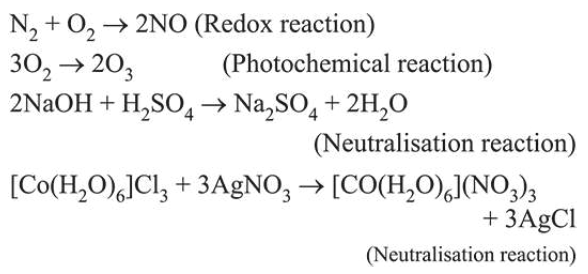# The redox reaction among the following is:Question:

The redox reaction among the following is:

1.  formation of ozone from atmospheric oxygen in the presence of sunlight

2. reaction of $\left[\mathrm{Co}\left(\mathrm{H}_{2} \mathrm{O}\right)_{6}\right] \mathrm{Cl}_{3}$ with $\mathrm{AgNO}_{3}$

3. reaction of $\mathrm{H}_{2} \mathrm{SO}_{4}$ with $\mathrm{NaOH}$

4.  combination of dinitrogen with dioxygen at $2000 \mathrm{~K}$

Correct Option: , 4

Solution: